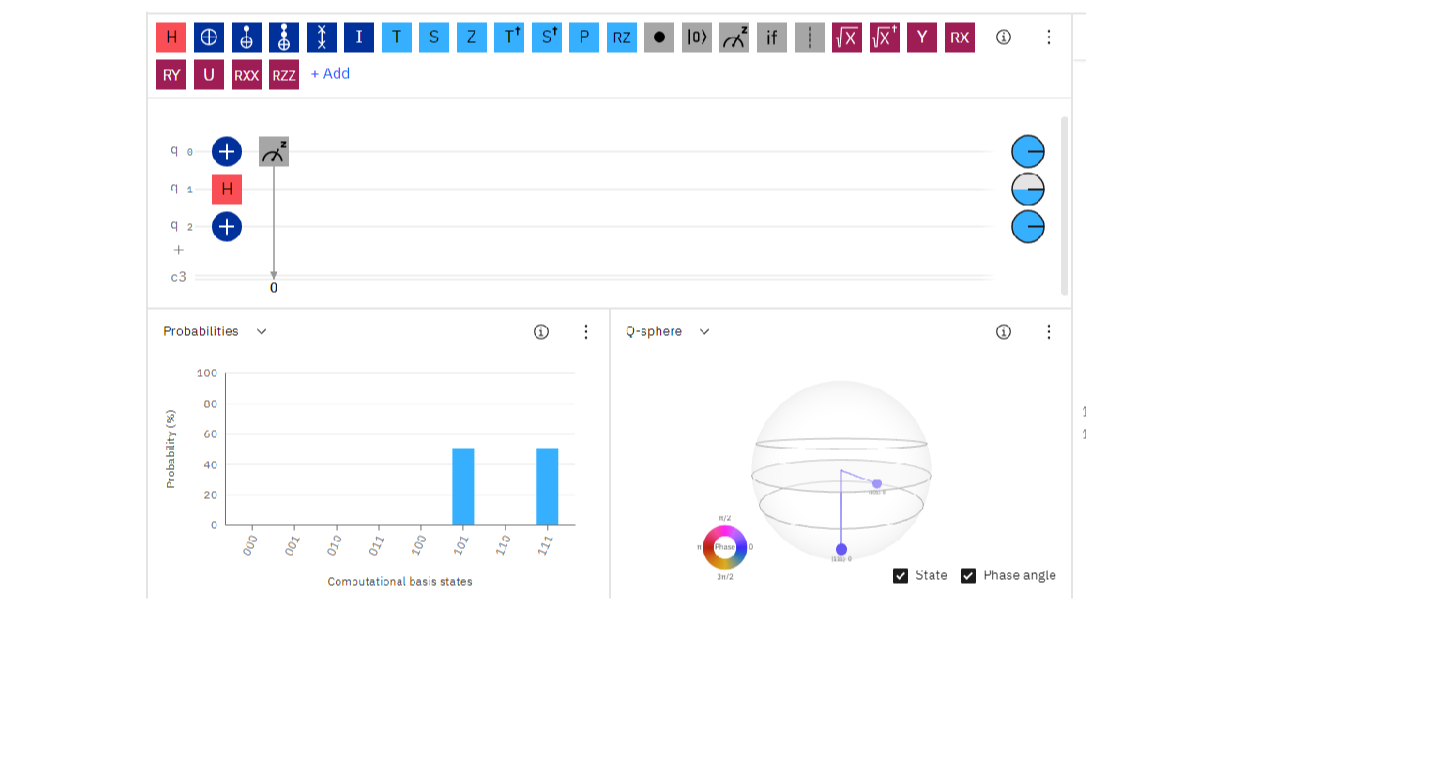Question

Python

3.Draw a quantum circuit with three qubits that returns|111i or |101i with50% probability eachVerified### Question 38860Python

8. Implement a solution to the Deutsch oracle problem as discussed in lectures in Qiskit (using the Deutsch-Jozsa Algorithm). (10 marks)

### Question 38859Python

7. Simulate a coin toss using qubit(s) in a quantum circuit.Why does this lead to better 'randomness' than a normal/classical coin toss? How could you extend this to be a random number generator? (3 marks)

### Question 38858Python

6. Make a quantum circuit whose final Bell state is 1 p2(|01i-|10i).

### Question 38857Python

5. What does the Bloch sphere represent? Describe the Bloch sphere created by the circuit in the previous question. (1 mark)

### Question 38856Python

4. Draw a quantum circuit with three qubits that returns|000i, |001i, |01Oi,|011i, |100i, |101i or |111i with equal probability.

### Question 38855Python

3.Draw a quantum circuit with three qubits that returns|111i or |101i with50% probability each

### Question 38854Python

2. Draw a simple quantum circuit with three qubits that returns |111i> with certainty.

### Question 38853Python

1.What does a Hadamard gate do?

### Question 29861Python

The following is an excerpt from the blink example:
Create a variable called time that will store the time intervals needed for the delay function. Rewrite the code above so that the variable is substituted for the value1000.
p) Create a second variable valued time2. Using time and time2, modify the code so that the LED stays on for 3 seconds, and off for 1.5 seconds.

### Question 29860Python

7. What is the difference between a variable and a constant?

### Submit query

Getting answers to your urgent problems is simple. Submit your query in the given box and get answers Instantly.

### Submit a new QuerySuccess

Assignment is successfully created